# Physics - Electromagnetism - Series R-L-C circuits Impedance## Introduction

Hello it'a me again @drifter1! Today we continue with the Electromagnetism series to talk about R-L-C circuit Impedance, which is the somewhat complex total Resistance of such circuits. To stay simple I will only cover Series circuits. To understand this post you should first check out the previous article about Electric Reactance.

So without further do, let's get started!

## Series R-L-C circuits

Circuits that contain a Resistor with Resistance R, Inductor with Inductance L and Capacitor with Capacitance C, connected in series across a voltage supply/source are called Series RLC circuits. We already covered some things about them in a "similar" article that you can find here. Now that we also know about Alternating current (AC) we can start talking about more stuff that is much more interesting.

The 3 elements that R-L-C circuits are build off are linear and passive in nature, which means that they consume energy rather than producing it. Therefore, these elements have a linear relationship between voltage and current that we covered last time in Reactance pretty well. We saw that there is a 90 degree Phase difference between the current and voltage waveforms for Capacitors and Inductors. For capacitors the current is leading over the voltage, whilst for inductors the voltage is leading over the current. The 90 degree angle can be explained very simple by thinking about the maximum and "zero" points of such graphs. When the voltage or current is at max, then the other quantity must be "zero" (small recap of last time).

These 3 components can be connected in many different ways. The simplest and most common ones are the series and parallel connection. What changes is the oscillation behavior and speed and that's why we will analyze only series R-L-C in depth, cause parallel ones are a little bit more complex! For series R-L-C circuits, like any other series connection, the current is the same for each element/component where current flows through [parallel circuits will have the same voltage].

By having an AC current the current through each component is: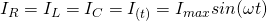The total voltage is not equal to a algebraic sum of voltages, but equal to a vector sum, because the voltage and current are not out-of-phase in the case of Capacitors and Inductors. Being a vector sum and knowing that current and voltage are in-phase for Resistors we therefore have:
where:

• Vs is the total voltage
• VR = I*R is the voltage across the resistor
• VL = I*XL is the voltage across the inductor
• VC = I*XC is the voltage across the capacitor

## Phasor Diagrams

Let's get more into the Phasor Diagrams of current and voltage over time to get into a proof of the previous equation!

As we already said before each element/component has a different phase "relationship" between the electric current and voltage. For resistors voltage and current are in-phase and so max out and "zero" at the same points. In the case of inductors the voltage leads over the current by 90 degrees, whilst for capacitors the current leads the voltage by 90 degrees.

An easy way of remembering the phase relationship is the word "CIVIL", which shows us that the current leads of the voltage in capacitors, whilst the voltage leads over the current in inductors.

For series circuits the current is the same and so what makes sense is the phase relationship between the "different" voltages. The current and voltage inside of a resistor are in-phase, which means that we can put it in the "center", along the same axis as the current phasor, which for example could be the positive x-axis direction. The inductor's voltage leads the current by 90 degrees and so we draw a vector perpendicular to the current phasor (or voltage vector of the resistor) "upwards". For the capacitor we do the same, but in the opposite direction ("downwards") cause the current leads the voltage by 90 degrees. Having three vectors the total vector sum of voltages contains 3 vectors that point in different directions. The vectors of the inductor and capacitor are on the same axis (y) and so we can say that the "partial" sum of them is: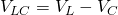Of course we are considering that the voltage across the inductor is larger then the voltage across the capacitor. To find the total sum we know only have to find the sum between two voltages using the Parallelogram rule of vector addition. Doing that we finally end up with:which is the same result that we got before.

## Impedance

Lastly, let's now also get into the Impedance or complex resistance of such circuits. As we saw in Reactance, Resistance is not the only cause of current limitation. Inductors and Capacitors also try to oppose the change in current through their ability called Reactance (inductive and capacitive). Acting in opposite ways, they partially to totally cancel each other's effect. As we saw last time the reactance of these components is frequency dependent and therefore the "total" Impedance of the circuit is also frequency dependent. The total resistance R stays the same, whilst the total reactance X = XL - XC changes and depends on the frequency/period of the AC current oscillation.

When having an Impedance or complex resistance that is being represented by 'Z' the AC version of Ohm's law now is:
We previously calculated the total voltage as a vector sum of the individual voltages across each component. Each voltage can be "replaced" by another expression that let's us make the expression of Impedance Z even simpler.

Step by step the Impedance of series R-L-C circuits comes out: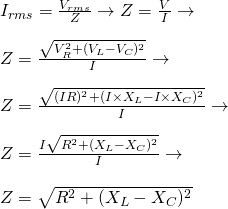For circuits without some of the 3 components we take out the corresponding part:

• R for circuits without resistor
• XL for circuits without inductor
• XC for circuits without capacitor

Another final thing that is worth noting is the series resonant condition:

• Z = R
• XC = XL
• ω = 1/sqrt(LC)
• Phase = φ = 0

that we will get into again later on in Resonance!

## Sum up

So, to sum up everything again:

• R-L-C circuits are circuits that consist of a resistance R, inductance L and capacitance C
• Series R-L-C circuits have the same current [I(t) = Isinωt] through each component/element
• Resistors only have a Resistance, whilst ideal Inductors and Capacitors have a property called Reactance (inductive and capacitive).
• The reactance of an inductor is X = ωL, whilst the reactance of an capacitor is X = 1/ωC
• The total reactance of a R-L-C circuit, considering zero reactance for ideal resistors is X = XL - XC
• The combined effect of resistance and reactance is called Impedance Z
• For series R-L-C circuits this Impedance is given by the equation: Z = sqrt[R^2 + (XL - XC)^2]

## REFERENCES:

Mathematical equations that I had in this post where drawn using quicklatex!

## Previous articles of the Electromagnetism series

Here we only have the previous two "chapters" and the current one....

### Electromagnetic Induction:

Motional Electromotive Force (Emf) -> Motional Emf, Faraday's law and motional emf, generalization

Lenz's law and Induced Electric fields -> Lenz's law, Induced Electric Fields

Eddy Currents and Applications -> Εddy currents, applications (brakes, testing, others)

Maxwell's equations -> What they are, each equation analyzed separately

Electromagnetic Induction exercises -> examples all around Electromagnetic Induction

### Mutual and Self Induction:

Mutual Inductance -> Mutual Inductance, applications

Self Induction -> Self Induction, Lenz's law, Inductance of a Coil

Magnetic Energy Density -> Energy stored in a magnetic field (or inductor), Magnetic energy density, Coaxial Cable Inductance Example

R-L circuits -> R-L circuit energizing, de-energizing, Characteristic time constant

L-C circuits -> L-C circuit, oscillations, energy cases, applications

R-L-C circuits -> R-L-C circuit, oscillation, applications

Mutual and Self Induction exercises -> examples all around Mutual and Self Induction

### Alternating current:

Getting into Alternating current -> Phasor diagrams, Alternating current, Average (RMS) current and voltage, Differences, Advantages/Disadvantages

Electric Reactance in AC circuits -> Resistors, Inductor and Capacitor Reactance in AC circuits

## Final words | Next time

And this is actually it for today's post! Next time we will get into the power/wattage of AC circuits and after that we will get into the Resonance of Series and Parallel R-L-C circuits, which will be a continuation to today's article!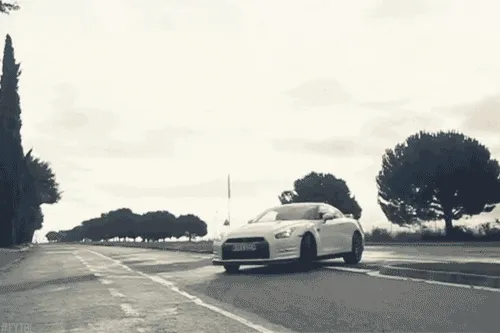Keep on drifting!

H2
H3
H4
3 columns
2 columns
1 column